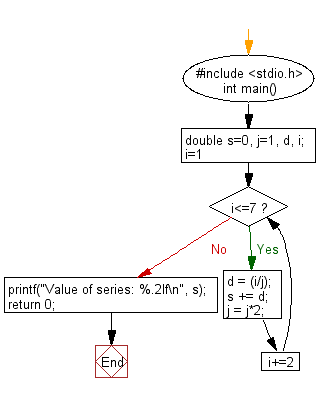﻿ C Program: Calculate the value of series of 4 numbers - w3resource

# C Exercises: Calculate the value of a series of 4 numbers

## C Basic Declarations and Expressions: Exercise-46 with Solution

Write a C program to calculate the value of S where S = 1 + 3/2 + 5/4 + 7/8.

Sample Solution:

C Code:

``````#include <stdio.h>
int main() {
double s=0, j=1, d, i;

// Loop to calculate the series
for(i=1; i<=7; i+=2){
d = (i/j);
s += d;
j = j*2;
}

// Print the result
printf("Value of series: %.2lf\n", s);

return 0;
}
``````

Sample Output:

```Value of series: 4.62
```

Flowchart:C programming Code Editor:

What is the difficulty level of this exercise?

Test your Programming skills with w3resource's quiz.

﻿